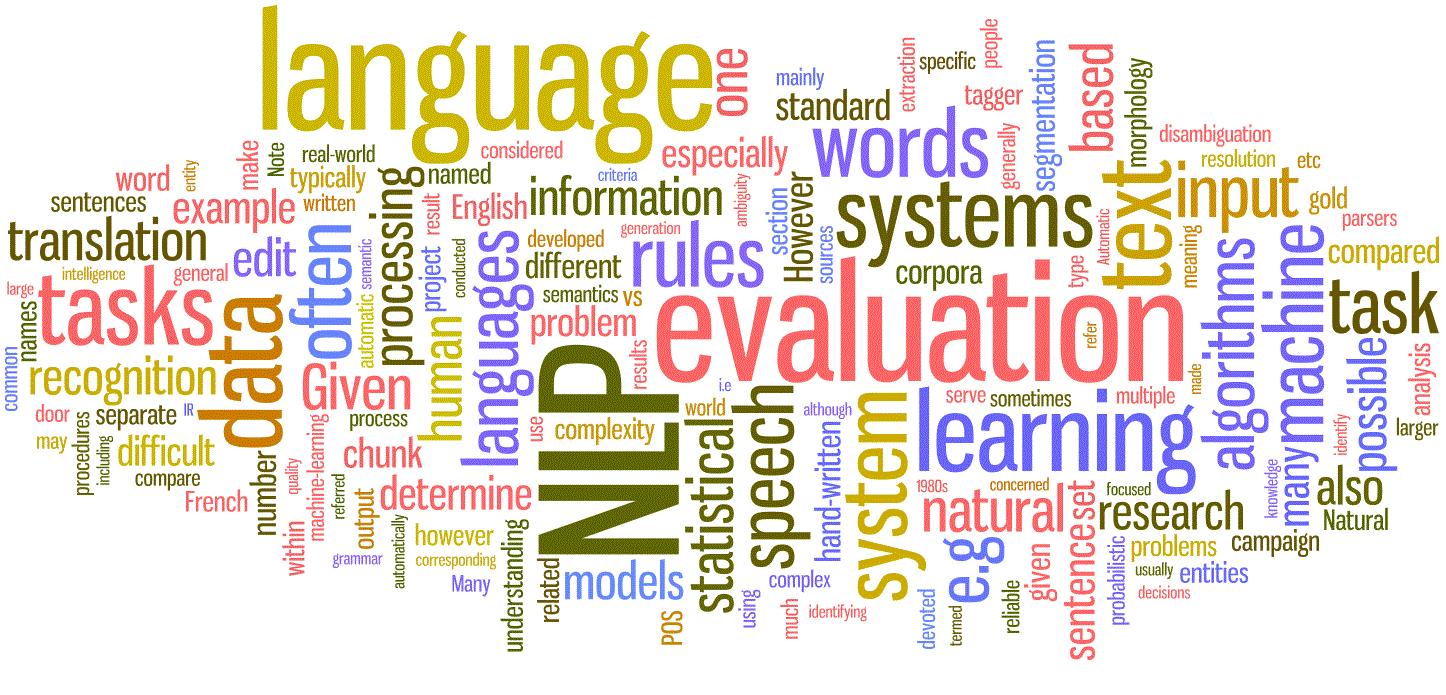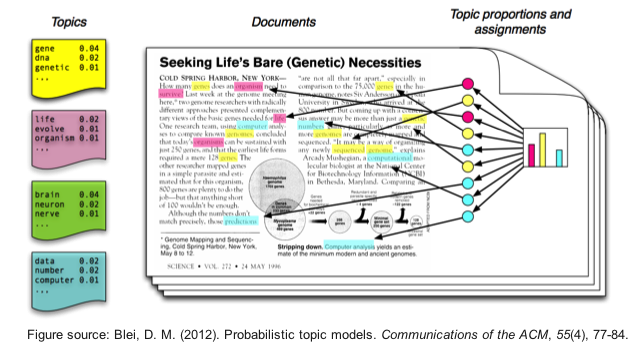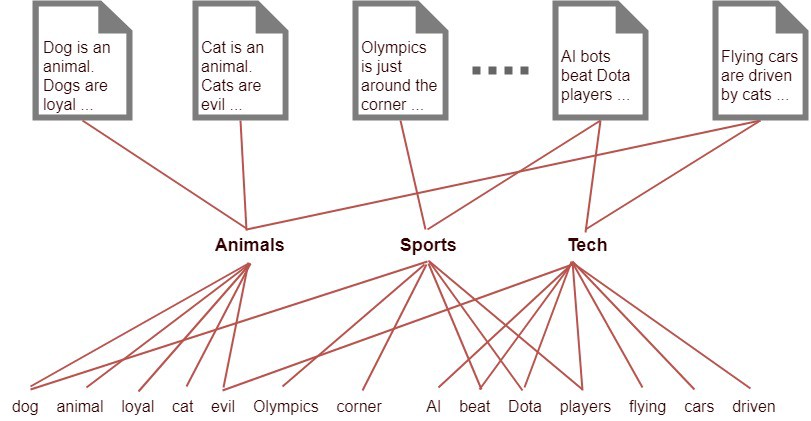×

Search anything:

# Topic Modelling Techniques in NLP

#### Machine Learning (ML) Natural Language Processing (NLP)In this article, we will look at what is actually mean by topic modeling, how it works and why it is necessary. We will also look into the important Topic Modeling techniques in the industry. Finally, we will see some of the real time applications and use cases of these techniques. The article is accompanied by coding snippets for easier understanding of these methods.# Introduction

Topic modeling is an algorithm for extracting the topic or topics for a collection of documents. It is the widely used text mining method in Natural Language Processing to gain insights about the text documents. The algorithm is analogous to dimensionality reduction techniques used for numerical data.

It can be considered as the process of obtaining required features from the bag of words. This is highly important because in NLP each word present in the corpus is considered as a feature. Thus feature reduction helps us focusing on the right content instead of wasting our time going through all the text in the data. For better understanding of the concepts, let us stay away from the mathematics background.## Question

#### This method is similar to which of the following techniques

Principal Component Analysis (PCA)
Long Short Term Memory (LSTM)
Recurrent Neural Network (RNN)
Bag of Words
Principal Component Analysis is the process of reducing the dimensionality of a very large matrix to a comparatively very small matrix. It removes the entries of the matrix with less relevance.

# Different Methods of Topic Modeling

This highly important process can be performed by various algorithms or methods. Some of them are:

• Latent Dirichlet Allocation (LDA)
• Non Negative Matrix Factorization (NMF)
• Latent Semantic Analysis (LSA)
• Parallel Latent Dirichlet Allocation (PLDA)
• Pachinko Allocation Model (PAM)

Still there are many research going on to improve the algorithms to understand the complete context of the documents.

## 01. Latent Dirirchlet Allocation (LDA)

Latent Dirichlet Allocation is a statistical and graphical model which are used to obtain relationships between multiple documents in a corpus. It is developed using Variational Exception Maximization (VEM) algorithm for obtaining the maximum likelihood estimate from the whole corpus of text. Traditionally, this can be solved by picking out the top few words in the bag of words. However this completely lack the semantics in the sentence. This model follows the concept that each document can be described by the probabilistic distribution of topics and each topic can be described by the probabilistic distribution of words. Thus we can get a much clearer vision about how the topics are connected.

For example, consider you have a corpus of 1000 documents. After preprocessing the corpus, the bag of words consists of 1000 common words. By applying LDA, we can determine the topics which are related to each document. Thus it is made simple to obtain the extracts from the corpus of data.In the above picture, the upper level represents the documents, the middle level represents the topics generated and the lower level represents the words. Thus it clearly explains the rule it follows that document is described a the distribution of topics and topics are described as the distribution of words.

The python implementation of this method is given below. Please give a hands on try to understand this completely. The data cleaning and text preprocessing part is not covered in this article.

``````from sklearn.feature_extraction.text import CountVectorizer
from sklearn.decomposition import LatentDirichletAllocation as LDA

count_vectorizer = CountVectorizer(stop_words='english')
count_data = count_vectorizer.fit_transform(papers['preprocessed_text'])

number_topics = 5

lda = LDA(n_components=number_topics)
lda.fit(count_data)
``````

Here, the parameter number_topics is completely dependent on the context and the requirement. If the value is very high, then more topics will be created might become difficult to obtain the insights. If the value is very less, then very few topics would be created and we might not get enough insights from the data.

## 02. Latent Semantic Analysis

Latent Semantic Analysis is also an unsupervised learning method used to extract relationship between different words in a pile of documents. This aids us in choosing the correct documents required. It simply acts as a dimensionality method used to reduce the dimension of the huge corpus of text data. These unnecessary data acts as a noise in determining the correct insights from the data.

``````from gensim import corpora
from gensim.models import LsiModel

def create_gensim_lsa_model(doc_clean,number_of_topics,words):
lsamodel = LsiModel(doc_term_matrix, num_topics=number_of_topics)
print(lsamodel.print_topics(num_topics=number_of_topics, num_words=words))
return lsamodel

number_of_topics=6
words=10
model=create_gensim_lsa_model(clean_text,number_of_topics,words)
``````

Here also the parameter number of topics play an important role. It is an iterative process to determine the optimum number of topics.

## 03. Non Negative Matrix Factorization

NMF is a matrix factorization method where we make sure that the elements of the factorized matrices are non-negative. Consider the document-term matrix obtained from a corpus after removing the stopwords. The matrix can be factorized into two matrices term-topic matrix and topic-document matrix. There are many optimization models to perform the matrix factorization. Hierarchical Alternating Least Square is a faster and better way to perform NMF. Here the factorization occurs by updating one column at a time while keeping the other columns as constant.

``````from sklearn.feature_extraction.text import TfidfVectorizer

from sklearn.decomposition import NMF

vectorizer = TfidfVectorizer(max_features=2000, min_df=10, stop_words='english')

vectorized_data = vectorizer.fit_transform(data)

nmf = NMF(n_components=20, solver="mu")

W = nmf.fit_transform(vectorized_data)
``````

## 04. Parallel Latent Dirirchlet Allocation

It is also known as Partially Labeled Dirichlet Allocation. Here, the model assumes that there exists a set of n labels and each of these labels are associates with each topics of the given corpus. Then the individual topics are represented as the probabilistic distribution of the whole of corpus similar to the LDA. Optionally, there could also be a global topic assigned to every document such that there are l global topics where l is the number of individual documents in the corpus. The method also assumes that there exists only one label for every topic in the corpus. With the labels given before developing the model, this process is very quick and precise compared to the above methods.

## 05. Pachinko Allocation Model

Pachinko Allocation Model (PAM) is an improved method of Latent Dirichlet Allocation model. LDA model brings out the correlation between words by identifying topics based on the thematic relationships between words present in the corpus. But PAM improvises by modeling correlation between the generated topics. This model has greater power in determining the semantic relationship precisely as they also take into account of the relation between topics. The model is named after Pachinko, a popular game in Japan. The model makes use of Directed Acrylic Graphs to understand the correlation between topics. DAG is a finite directed graph to show how the topics are related.

# Applications

• Topic modeling can be used in graph based models to obtain semantic relationship between words.
• It can be used in text summarization to quickly find out what the document or book is explaining about.
• It can be used in exam evaluation to avoid biasing towards candidates. It also saves a lot of time and helps students get their results quickly.
• It can provide improved customer service by identifying the keyword the customer is asking about and acting accordingly. This increases the trust of customers as they received the help needed at the right time without any inconvenience. This drastically improves the customer loyalty and in turn increases the value of the company.
• It can identify the keywords of search and recommend products to the customers accordingly.

#### Which of the following is not an application of Topic Modeling?

Time Series Analysis
Recommender Systems
Healthcare Systems
Text Summarization
Principal Component Analysis is the process of reducing the dimensionality of a very large matrix to a comparatively very small matrix. It removes the entries of the matrix with less relevance.

# Conclusion

Thus both of these methods aid us in getting the correct information from the data we provide. It keeps us focused on the correct portion data by removing unnecessary data from the corpus. These methods are highly useful in obtaining the business value from the data.#### Murugesh Manthiramoorthi

Data Scientist with 1.5 years of experience

Topic Modelling Techniques in NLP